# TRIM – Part II THE SEQUEL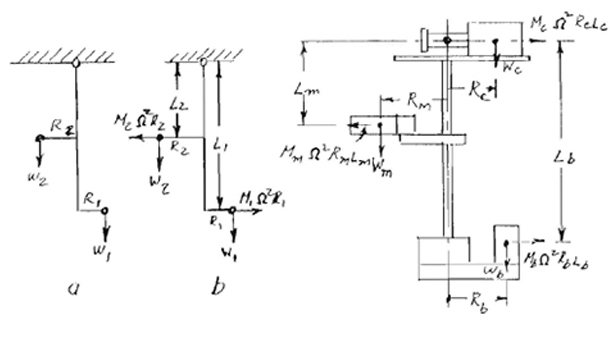Steadicam letter Volume 1, Number 2 (1988)

The Grand Unified Theory of Trim
by Arnold DiGiulio

Trimming Steadicam for proper panning is essentially a problem in dynamic balance, not unlike that of balancing a wheel. Two salient differences mask the similarity. First, since at highway speeds a wheel rotates much more rapidly than Steadicam, even during whippanning, the reactions are much more violent.

Second, since the wheel is mounted in a rigid bearing, all it can do to protest its unhappy state is vibrate. In the case of Steadicam, however, the bearing is no more rigid than the operator’s hand, and panning is a compromise between the will of the operator and the demands of physics.

In order to understand what happens, a short theoretical review is in order. Dynamic imbalance is caused by centrifugal forces. Masses deployed about an axis act on it only by their weight as long as they are stationary. Thus if they are in equilibrium, they are statically balanced. If these masses are deployed in a single plane perpendicular to the axis (fig. 1), they are also dynamically balanced. But if the masses are not coplanar, when the axis is rotated, (unequal) centrifugal forces will cause the axis itself to move about an entirely different axis. (Note: “coplanar” here means that all the masses are in the same horizontal plane such as if the monitor could be lowered enough to be at the same level as the battery and electronics. With the monitor raised (as it is on the Model III and Model II) we have dynamic imbalance.)

The expression for centrifugal is:
(1) F=MΩ²R
Where F is the centrifugal force
M is the mass (which is directly proportional to the weight)
Q (Omega) is the angular velocity (a common unit for this is RPM)
R is the distance from the axis of rotation to the center of mass (i.e. the CO of the mass)

By way of explanation, I will briefly discuss the theory of dynamic balance and illustrate it with a simple problem. Then I will discuss a technique that does not involve mathematics.

Let us consider a lightweight rod held at one end but free to swing (fig. 2) and suspended vertically. The rod has two forces F₁ and F₂ acting on it as shown. The rod will remain vertical as long as F₁L₁ = F₂L₂ (i.e. the area A₁ & A₂ are equal). Note that I said forces and not weights.

Now let ‘s replace those forces with weights (fig. 3). In simple static balancing, the rod will remain vertical if W₁R₁ = W₂R₂.

Now let’s spin the rod (fig. 3). The rod will not spin about its axis; the lower end of the rod will describe a circle. The reason that this happens is that M₂ΩR₂L₂ is less than M₁ΩR₁L₁ because L₂ is less than L₁.

The system is not in dynamic balance.

Dynamic balance can be achieved by the condition shown in the following equation:

(2) M₂Ω²R₂L₂ + R₁W₁ = M₁Ω²R₁L₁ + R₂W₂

Note: the sum of forces that tend to rotate the figure counterclockwise equals the sum of forces which tend to rotate it clockwise)

Note that I have stated that M is directly proportional to W, but it is not the same number. At sea level using the foot, pound, second system of units, W is 32.17M

You will note from the foregoing equation that R₂W₂ must be greater than R₁W₁. The system may be balanced statically or dynamically but not both. This happens because part of the force to achieve dynamic balance is supplied by whatever is holding the rod. In the case of Steadicam, which is a three mass system, both static and dynamic balance are achieved.

Now let’s look at Steadicam (fig. 4). Assume a point on a horizontal plane through the CO of the camera and on the axis of the centerpost. Call this the reaction point as shown in figure 4. (Arnold is referring to the point shown within the lens in fig. 4) Let us further assume that when dynamic balance is achieved, static balance is also achieved. This will be illustrated later.

Forces acting through a distance so as to cause rotation are called moments (not momentum). Thus the quantities in equation (2) are moments. Let’s adopt the convention that a moment that tends in the counterclockwise direction is positive, one in the clockwise direction is negative.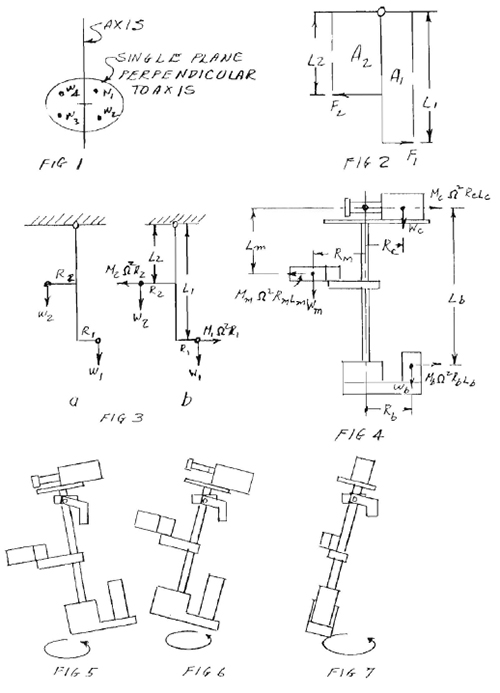When the sum of all the moments in a system is equal to zero the system is in equilibrium. If the moments due to Q are included it is in dynamic equilibrium.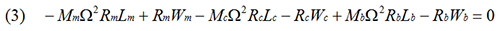Now if the system is in static equilibrium the static terms must equal zero.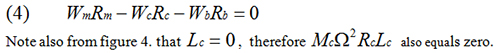These combinations that result in zero can be dropped from the equation. Thus: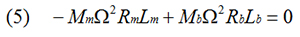We said that mass is directly proportional to weight, therefore we can substitute weight for mass since it affects both terms equally. Furthermore since Ω² appears in both terms, it can be eliminated.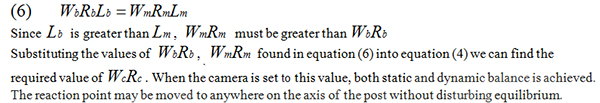To illustrate let’s assign some arbitrary numbers.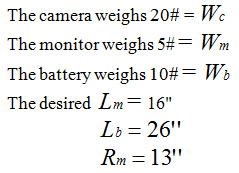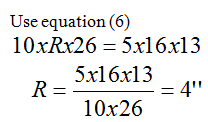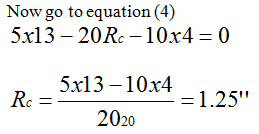By setting Rc to 1.25 we have achieved static equilibrium. Therefore the static terms can be eliminated from equation (3).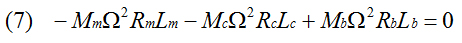Again, since the Ω² and M terms appear in each term we can eliminate the Ω² and use W for M.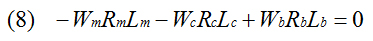Let’s move the reaction point down six inches. Then: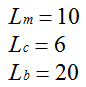Substituting in equation (8)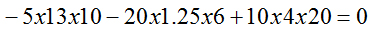So the system is indeed in dynamic and static equilibrium. If we set the system up like this it might need some fine tuning because we haven’t accounted for some minor weights such as the bar that carries the monitor or the electronics package which is more or less centered on the post.

The non-mathematical approach is based on the same principle as the theory but it is done with the unit in a trial and error fashion. First balance the system statically, placing the monitor where it is desired. Clamp the gimbal to some rigid object. there should be sufficient clearance for the unit to swing through 360°.

Spin the center post. Do this carefully since your hand may introduce extraneous motions. A good way to do this is to start the unit rotating slowly and then build up the speed by additional twists. Observe the bottom of the post.

If the system is not dynamically balanced the bottom of the post will start moving in a small circle. If the battery is to the outside of the circle as in figure 5, the battery is too far from the post. Move the battery closer to the post and rebalance by moving the camera away.

This condition can also be corrected by moving the monitor away from the post and rebalancing with the camera or by lowering the monitor.

If the monitor is to the outside of the circle as in figure 6, the battery is too close to the post. Move the battery away and rebalance with the camera. This condition can also be corrected by moving the monitor closer to the post and rebalancing with the camera or by raising the monitor.

A third condition can occur. Both battery and monitor are seen as tangential to the circle as in figure 7. This happens when the three masses are not in the same plane with the axis. In order to balance a three mass system you must have all masses in a common plane with the axis. To correct this move either the battery or the monitor toward the circle. Once you have achieved dynamic balance, you may move the gimbal anywhere along the post. Dynamic balance will not be affected.

This concludes Arnold Digiulio’s treatise on the mathematics of trim. Even if you end up using only one of the empirical methods in real life, it is probably useful to study and understand the physics involved.